Function Repository Resource:

# UnsortedComplement

Delete the elements of some lists from a list x without changing either the order of x or the multiplicities of its elements

Contributed by: George Beck
 ResourceFunction["UnsortedComplement"][list, del1,del2, …] deletes from listany occurrence of an element of the del1,del2,… without otherwise changing list.

## Details and Options

ResourceFunction["UnsortedComplement"] differs from Complement, which deletes but also reduces the multiplicity of each element to one and sorts.
ResourceFunction["UnsortedComplement"] accepts SameTest as an option.

## Examples

### Basic Examples (1)

Delete specified elements from a List:

 In:=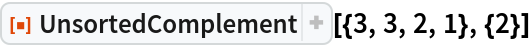Out=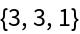### Scope (1)

Deleting with several lists is equivalent to deleting with their Join:

 In:=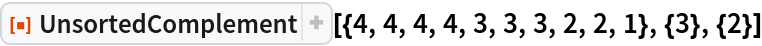Out=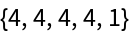In:=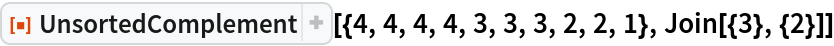Out=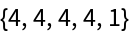### Options (2)

Use equivalence classes based on absolute value:

 In:=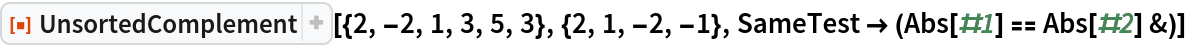Out=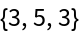This considers elements the same if they are identical modulo 3:

 In:=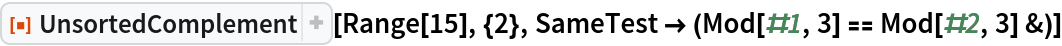Out=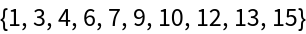These are the deleted elements:

 In:=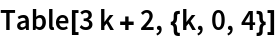Out=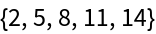Here there is no second list, so nothing is deleted:

 In:=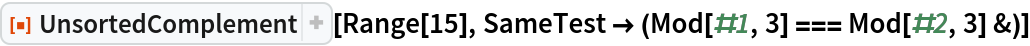Out=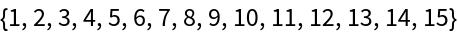### Properties and Relations (2)

Complement deletes elements, reduces remaining elements to one occurrence of each, and sorts:

 In:=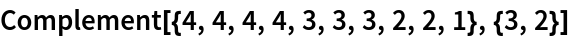Out=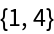UnsortedComplement only deletes elements:

 In:=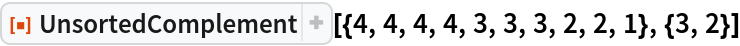Out=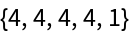Make two lists:

 In:=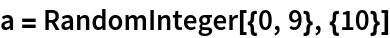Out=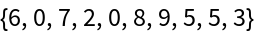In:=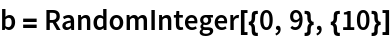Out=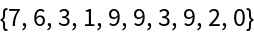Union deletes duplicates and sorts:

 In:=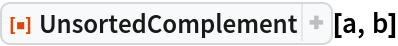Out=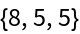In:=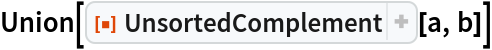Out=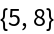In:=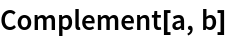Out=George Beck

## Requirements

Wolfram Language 11.3 (March 2018) or above

## Version History

• 1.0.0 – 12 February 2019